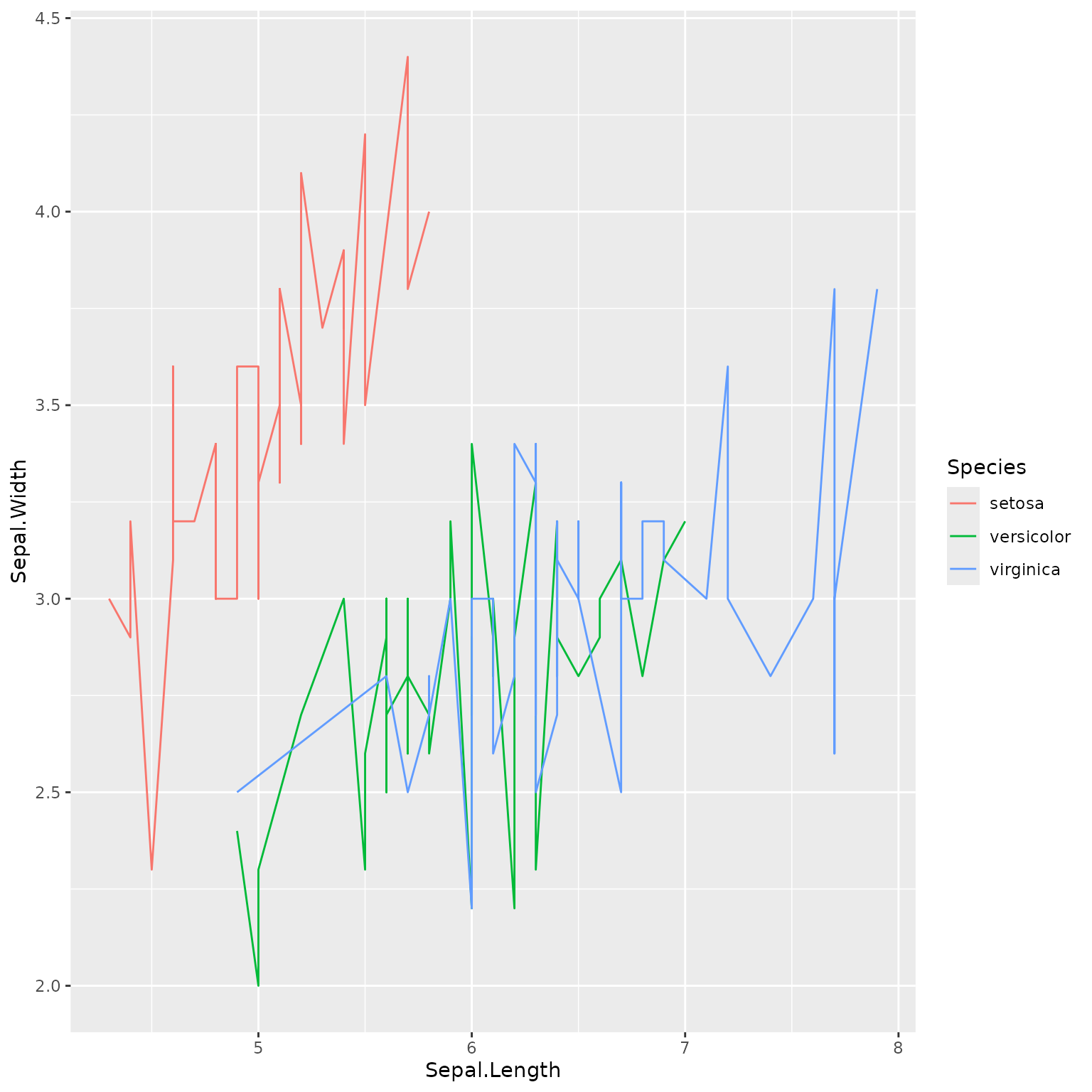This package allows easy access to some common ggplot2 tasks.

library(ggplot2)
library(patchwork)
library(labelled)
library(ggeasy)

## Rotate Plot Labels

Rotating the x axis labels is a very frequently looked up task, and we can make it easier. If we create a simple ggplot2 plot

p <- ggplot(mtcars, aes(hp, mpg)) + geom_point()

then by default, this looks like

p + labs(title = "ggplot2 default")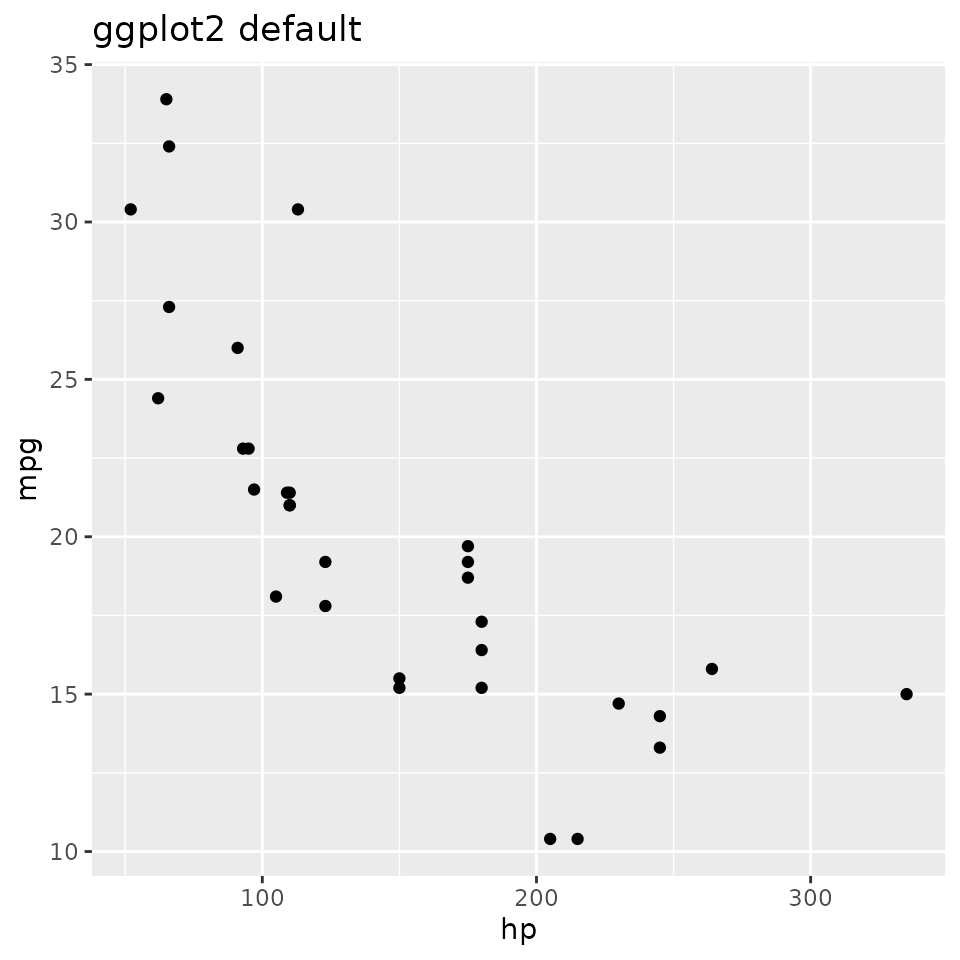We can perform various rotations though

p1 <- p +
easy_rotate_x_labels() +
labs(title = "default rotation")
p2 <- p +
easy_rotate_x_labels(angle = 45, side = "right") +
labs(title = "angle = 45")
p3 <- p +
easy_rotate_x_labels("startattop") +
labs(title = "text starts at top")
p4 <- p +
easy_rotate_x_labels("startatbottom") +
labs(title = "text starts at bottom")

(p1 + p2) / (p3 + p4)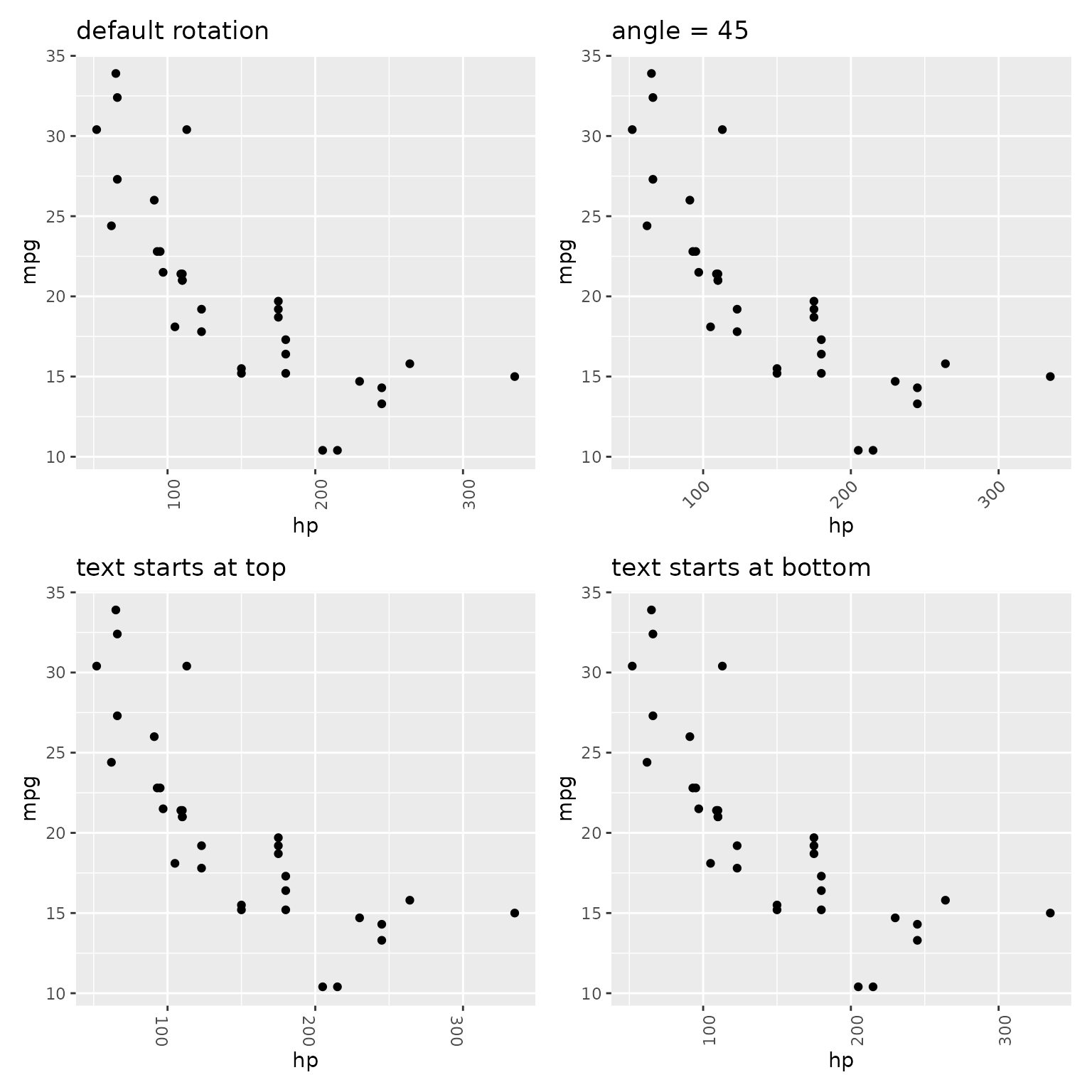## Removing legends

Removing legends is made easier by the easy_remove_legend function. When called without arguments, all legends are removed (equivalent to theme(legend.position = "none")). Alternatively, the names of aesthetics for which legends should be removed can be passed.

p <- ggplot(mtcars, aes(wt, mpg, colour = cyl, size = hp)) +
geom_point()

p1 <- p +
labs(title = "With all legends")
p2 <- p +
easy_remove_legend() +
labs(title = "Remove all legends")
p3 <- p +
easy_remove_legend(size) +
labs(title = "Remove size legend")
p4 <- p +
easy_remove_legend(size, color) +
labs(title = "Remove both legends specifically")

(p1 + p2) / (p3 + p4)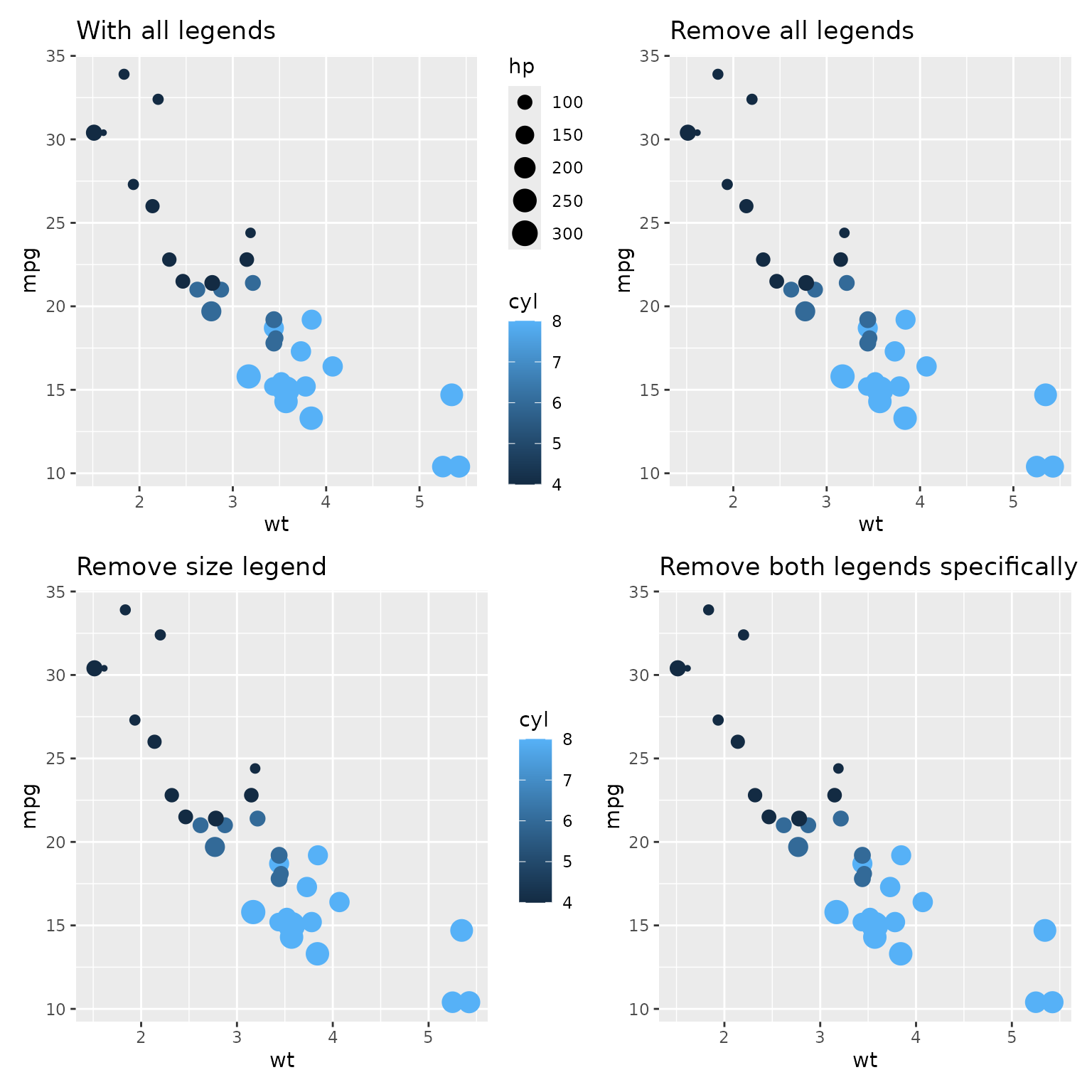## Plot Labels

Changing plot labels to a specified string isn’t particularly difficult (labs(x = "my label")) but wouldn’t it be even nicer if you could just add labels to your data.frame columns (e.g. using labelled::var_labels()) and have these reflected in your plot. easy_labs() makes this possible.

## create a copy of the iris data
iris_labs <- iris

## add labels to the columns
lbl <- c('Sepal Length', 'Sepal Width', 'Petal Length', 'Petal Width', 'Flower\nSpecies')
var_label(iris_labs) <- split(lbl, names(iris_labs))

These are visible if you use View(iris_labs) in RStudio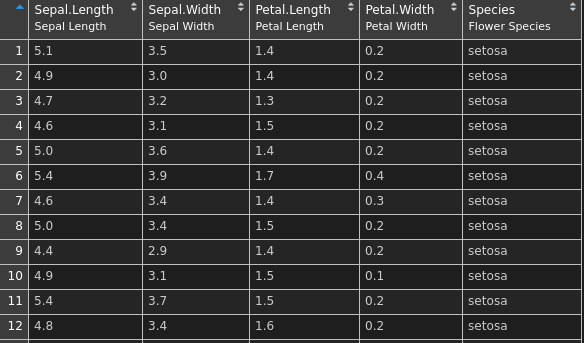p <- ggplot(iris_labs, aes(x = Sepal.Length, y = Sepal.Width)) +
geom_line(aes(colour = Species))

p1 <- p + labs(title = "default labels")
p2 <- p +
easy_labs() +
labs(title = "Replace titles with column labels")
p3 <- p +
easy_labs(x = 'My x axis') +
labs(title = "Manually add x axis label")

iris_labs_2 <- iris_labs
var_label(iris_labs_2\$Species) <- "Sub-genera"

p4 <- p + geom_point(data = iris_labs_2, aes(fill = Species), shape = 24) +
easy_labs() +
(p1 + p2) / (p3 + p4)p4 + geom_point(data = iris_labs_2, aes(fill = Species), shape = 24) +
labs(title = "Facetting works")